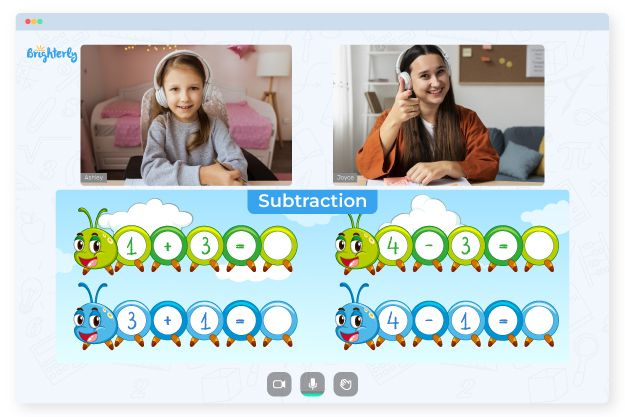# Subtracting Integers Worksheets

In grade school, kids learn topics on integers, one of them being how to subtract integers. Learning to subtract integers may require an out-of-the-box approach like worksheets. With a subtraction of integers worksheet, you can help your kids master integers with relative ease.

## Free subtracting negative integers worksheets

If you subtract two numbers, often the result is a smaller number. On the other hand, integer subtraction can result in either an increase or a reduction in the value of the original number. This logic may be challenging for kids to comprehend, hence the importance of worksheets.

Subtracting integers worksheets help kids understand why it’s vital to practice subtraction of integers. As your kids practice how to solve the problems in the sheet, they will learn to reason logically and advance in their understanding of arithmetic and the world.

Math for Kids

Is Your Child Struggling With Math?
1:1 Online Math TutoringWorksheets for teaching children how to subtract integers are a great way to help them grasp the subject and develop their logical reasoning skills.

Brighterly’s worksheets follow the widespread belief that the best form of teaching is to start from the base. Rather than overwhelming kids with complex questions, which could be counterproductive, Brighterly adopts a stepwise learning format. Kids will start from the simple integers subtraction before proceeding to the complex ones.

The designs and questions of the worksheets differ depending on the grade level. Subtracting integers worksheet grade 7 kids use will be more challenging than lower-level resources. On the other hand, integers worksheets for first graders will be more colorful and catchy.

## Printable subtracting integers worksheet PDF### Subtracting Integers Worksheets PDF

Subtracting Integers Worksheet### Subtracting Integers Worksheets PDF

Subtracting Integers Worksheet PDF### Subtracting Integers Worksheets PDF

Subtracting Integers Worksheet With Answers PDF### Subtracting Integers Worksheets PDF

Subtracting Negative Integers Worksheets

Subtracting integers worksheets can be a valuable tool for educators and parents seeking to improve their kids’ knowledge of the subject. The use of these tools reduces the time used in teaching significantly. For instance, instead of taking a week for kids to master the concept without a worksheet, it could take 2 to 3 days for them to understand it using engaging sheets. With a printable version available, they will have enough time to repeatedly solve the problem on the sheet.

Using the printable subtracting integers worksheet with answers PDF, you can teach your kids to study independently. Through the answers in the worksheet, they will know when they are wrong and correct themselves, which eventually leads to the mastery of the topic.

### More Integers Worksheets

Problems with Subtraction?• Is your child finding it hard to understand subtraction?
• An online tutor could be of help.

Does your child struggle with mastering subtraction? Try lessons with an online tutor.